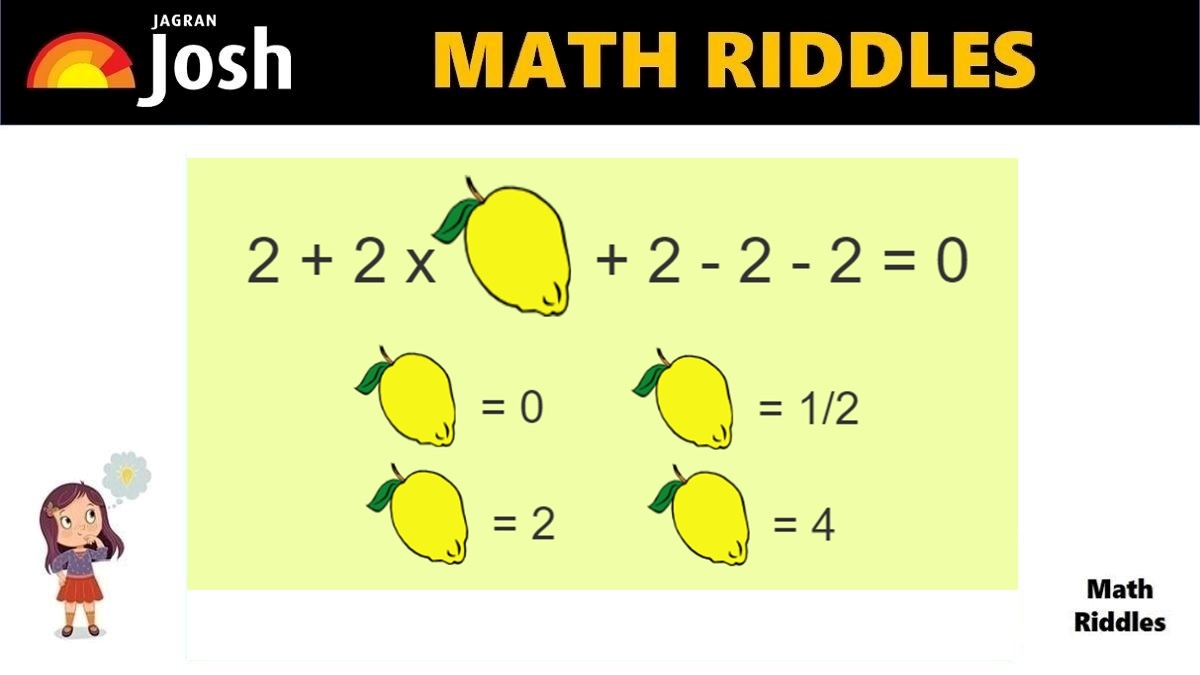# Math Riddles With Answers: Can You Find The Value Of Lemon In 20 Seconds?

Are you a math genius? Only 1% with a high IQ can solve this tricky Rebus math puzzle in 20 seconds.Math Riddles With Answers: Can You Find The Value Of Lemon In 20 Seconds?

Try to solve this Math Puzzle within 20 seconds. Math enthusiasts who love solving tricky math puzzles and arithmetic problems are big fan of Math Puzzles. You need high level of logical reasoning and mathematical knowledge. This math puzzle is a kind of brain teaser which can very cleverly hide a numerical expression. In this math puzzle, you need to find the value of lemon so that the equation stands true. The options for the right answer are given in the math picture puzzle, good luck finding the right answer.

If you love solving cryptic math puzzles, give this math puzzle a try! You have 20 seconds to find the correct answer.

## Math Riddles with Answers: Solution

Explanation:

This is a Math Puzzle in which the arithmetic operations and numbers are encrypted with the help of pictures and words. They are presented in a mysterious confusing picture puzzle that can trick your brain in one go. One has to apply logical thinking to avoid getting tricked.

In this Math Riddle, if you just glance at the equation quickly to the equation, you will definitely make a mistake. 99% of people failed to find the right answer quickly in 11 seconds.

Math Riddles With Answers: Can You Find The Value of Each Candy in 20 Seconds?

Math Riddles With Answers: Can You Solve This Tricky Math Rebus Puzzle 20 Seconds?

Look carefully at the picture, the equation equals to 0. If you see the multiplier of the lemon in the equation, you will understand that the number to be multiplied has to give product 0 as when you add 2 + 2 and both negative 2’s you will 4 – 4 which equals to 0.

Hence, when you apply the value of lemon as 0 you will find the product to be 0.

Hence, the value of the lemon is 0.

## Tell us in comments: Did you solve this math puzzle correctly in 20 seconds?

Check out more amazing tricky math puzzles!

Math Riddles With Answers: Can You Find The Value of Burger, Fries, Coke in 20 Seconds?

Math Riddles With Answers: Can You Find The Value of Each Fruit in 20 Seconds?

Picture Puzzle: Find Two Same Emojis In 11 Seconds, 99% People Failed To Find Them

Picture Puzzle: Only 1% Genius Can Find The Hidden Words In The Picture in 11 seconds

Get the latest General Knowledge and Current Affairs from all over India and world for all competitive exams.
खेलें हर किस्म के रोमांच से भरपूर गेम्स सिर्फ़ जागरण प्ले पर